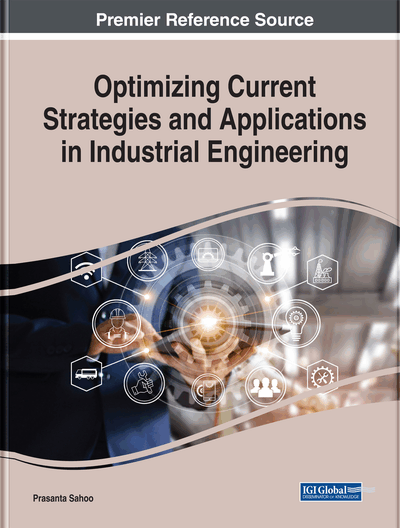# Linear Programming Based on Piece-Wise Linearization for Solving the Economic Load Dispatch Problem

Ahmad Al-Subhi (Yanbu Industrial College, Saudi Arabia) and Hesham K. Alfares (King Fahd University of Petroleum and Minerals, Saudi Arabia)
Copyright: © 2019 |Pages: 31
DOI: 10.4018/978-1-5225-8223-6.ch002
OnDemand PDF Download:
Available
\$37.50
No Current Special Offers

## Abstract

This chapter discusses the application of linear programming (LP) techniques to find the optimal solution of the economic dispatch (ED) problem without considering transmission losses. The ED problem is concerned with optimizing the power generated by several generating units. The objective is to find the optimal power produced by each unit to supply the required load at minimum total cost. The generation cost associated with each unit is usually in the form of a quadratic or cubic function of the power produced. To apply LP, these nonlinear cost functions have to be linearized. The optimal solution is then determined by LP based on the approximate linear model. Piece-wise linearization methodology is adopted in this chapter. To evaluate the performance of the linearization method, a comprehensive set of benchmark test problems is used. LP solutions of linearized ED problems are compared with several other techniques from the literature. The LP technique with piece-wise linearization shows an overall competitive advantage in terms of total cost, solution time, and load satisfaction.
Chapter Preview
Top

## Literature Review

Due to its practical and theoretical significance, the ED problem is an active area of continuing research. This is illustrated by a large number of ED papers that have been published in recent years. A recent literature search by the authors resulted in finding many works published on ED optimization using both classical and new methods. Some of the techniques used recently to solve ED problems include Mixed Integer Quadratic Programming (MIQP) (Absil et al., 2018), Gradient Method (Imen et al., 2013), Fast Lambda Iteration (FLA) (Zhan et al., 2014), Enhanced Lambda Iteration (ELI) (Singhal et al., 2014), and Artificial Neural Networks (ANN) (Momoh & Reddy, 2014).

Recent heuristic techniques used to solve the ED problem include PSO (Lin et al., 2015). PSO is one of the most commonly used heuristic algorithms used for ED applications. Abbas et al. (2017) perform a thorough literature survey of research papers that employed PSO to solve the ED problem. Other recently used heuristic techniques include Harmony Search (HS) algorithm (Al-Betar et al., 2018), Immune Log-Normal Evolutionary Programming (ILNEP) (Mansor & Musirin, 2017), Chaotic Bat Algorithm (CBA) (Adarsh et al., 2016), Ant Lion Optimization (ALO) (Nischal & Mehta, 2015), Gravitational Search Algorithm (GSA) (Hota & Sahu, 2015), Flower Pollination Algorithm (FPA) (Vijayaraj & Santhi, 2016), GA (Oluwadare et al., 2016), stochastic Whale Optimization Algorithm (Mohamed et al., 2018) and Grey Wolf Optimization (GWO) (Tung & Chakravorty, 2015).

## Complete Chapter List

Search this Book:
Reset